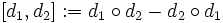# Lie ring of derivations

Let$L$ be a Lie ring. The Lie ring of derivations of$L$, denoted$\operatorname{Der}(L)$, is defined as a Lie ring whose elements are the derivations of$L$, where:
• The Lie bracket is given by the commutator. Thus, if$d_1,d_2:L \to L$ are derivations, their Lie bracket is defined as:$[d_1,d_2] := d_1 \circ d_2 - d_2 \circ d_1$.
In other words,$[d_1,d_2](x) = d_1(d_2(x)) - d_2(d_1(x))$.